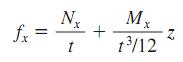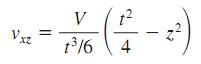Search

# Stresses In Thin Shells

Posted in Building |Email This Post |

Results of membrane and bending theories are expressed in terms of unit forces and unit moments, acting per unit of length over the thickness of the shell. To compute the unit stresses from these forces and moments, usual practice is to assume normal forces and shears to be uniformly distributed over the shell thickness and bending stresses to be linearly distributed. Then, normal stresses can be computed from equations of the form:where
z=distance from middle surface
t=shell thickness

Mx= unit bending moment about an axis parallel to direction of unit normal force Nx

Similarly, shearing stresses produced by central shears T and twisting moments D may be calculated from equations of the form:Normal shearing stresses may be computed on the assumption of a parabolic stress distribution over the shell thickness:where V=unit shear force normal to middle surface.

More Entries :